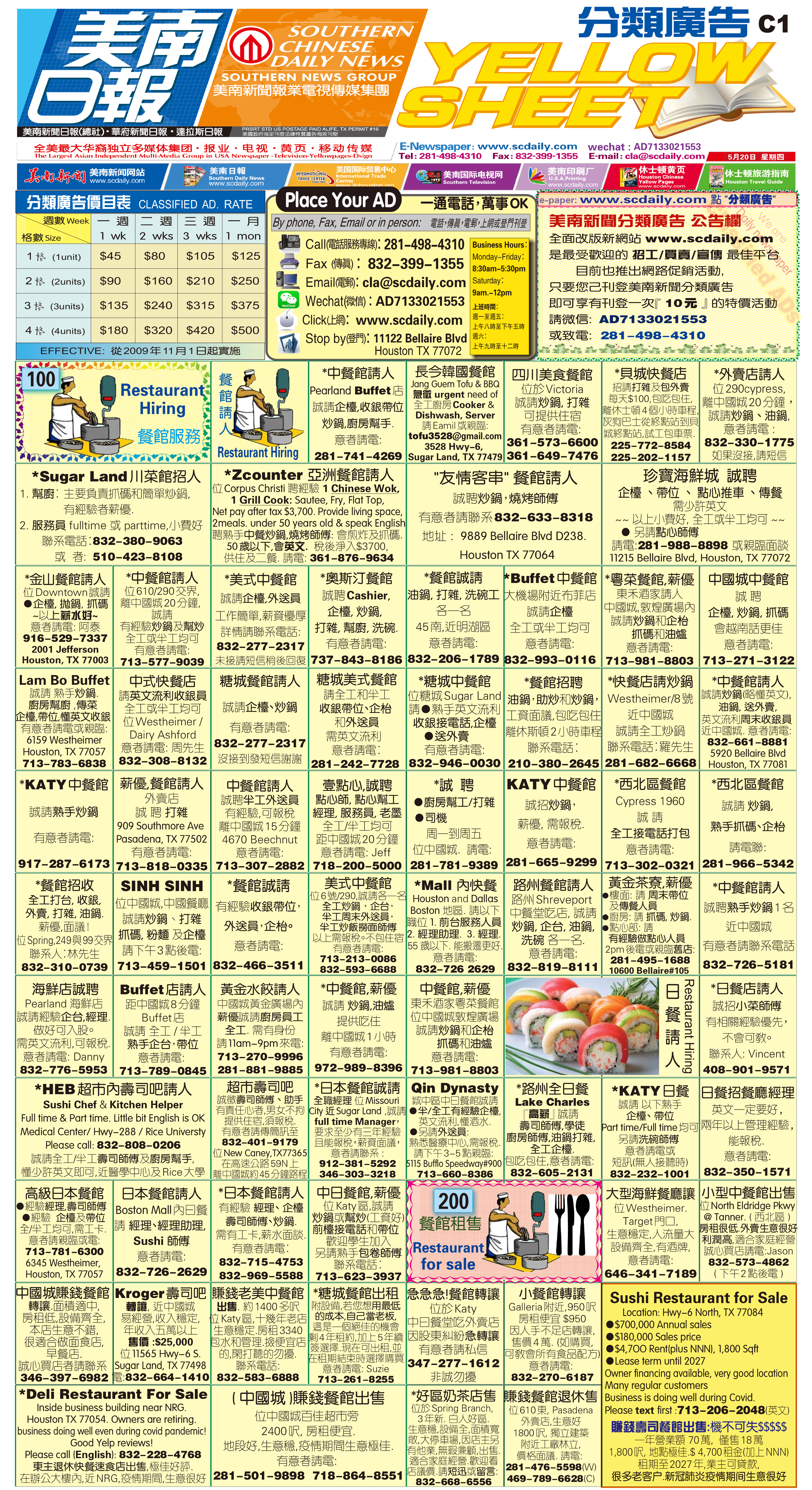210530 Epaper

 A Section B SectionC Section D Section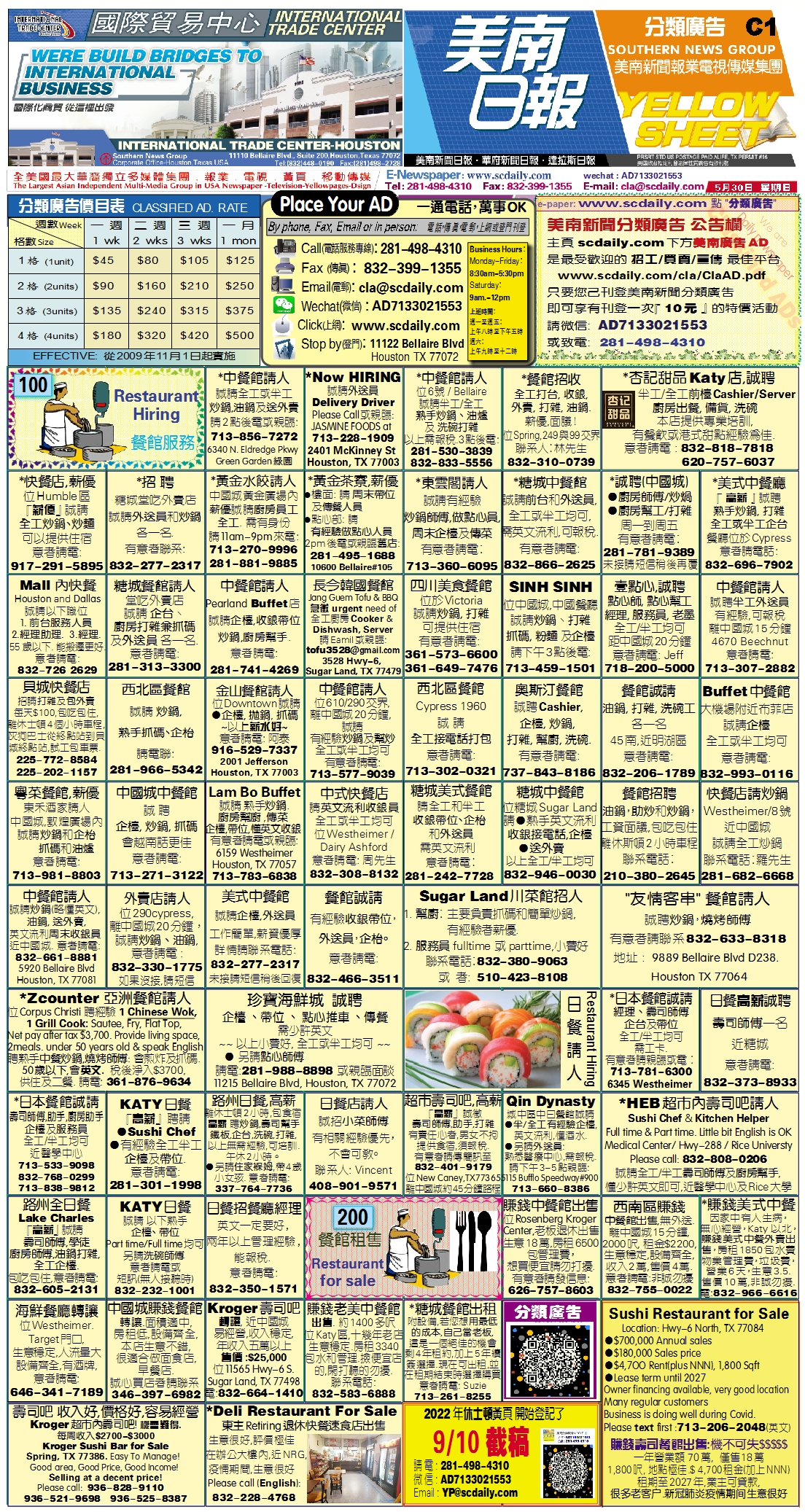210529 Epaper

 A Section B SectionC Section D Section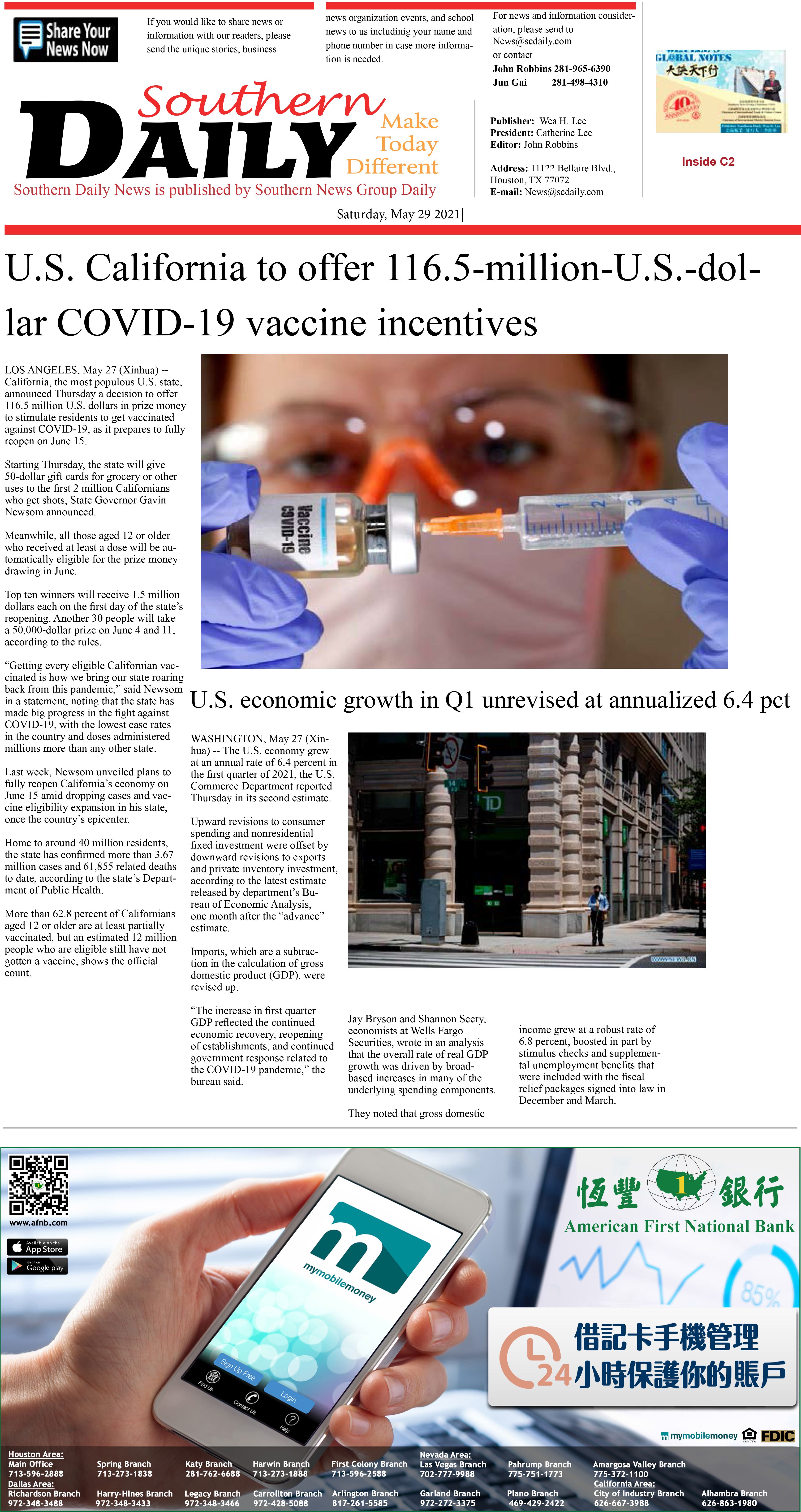210528 Epaper

 A Section B SectionC Section D Section210527 Epaper

 A Section B SectionC Section D Section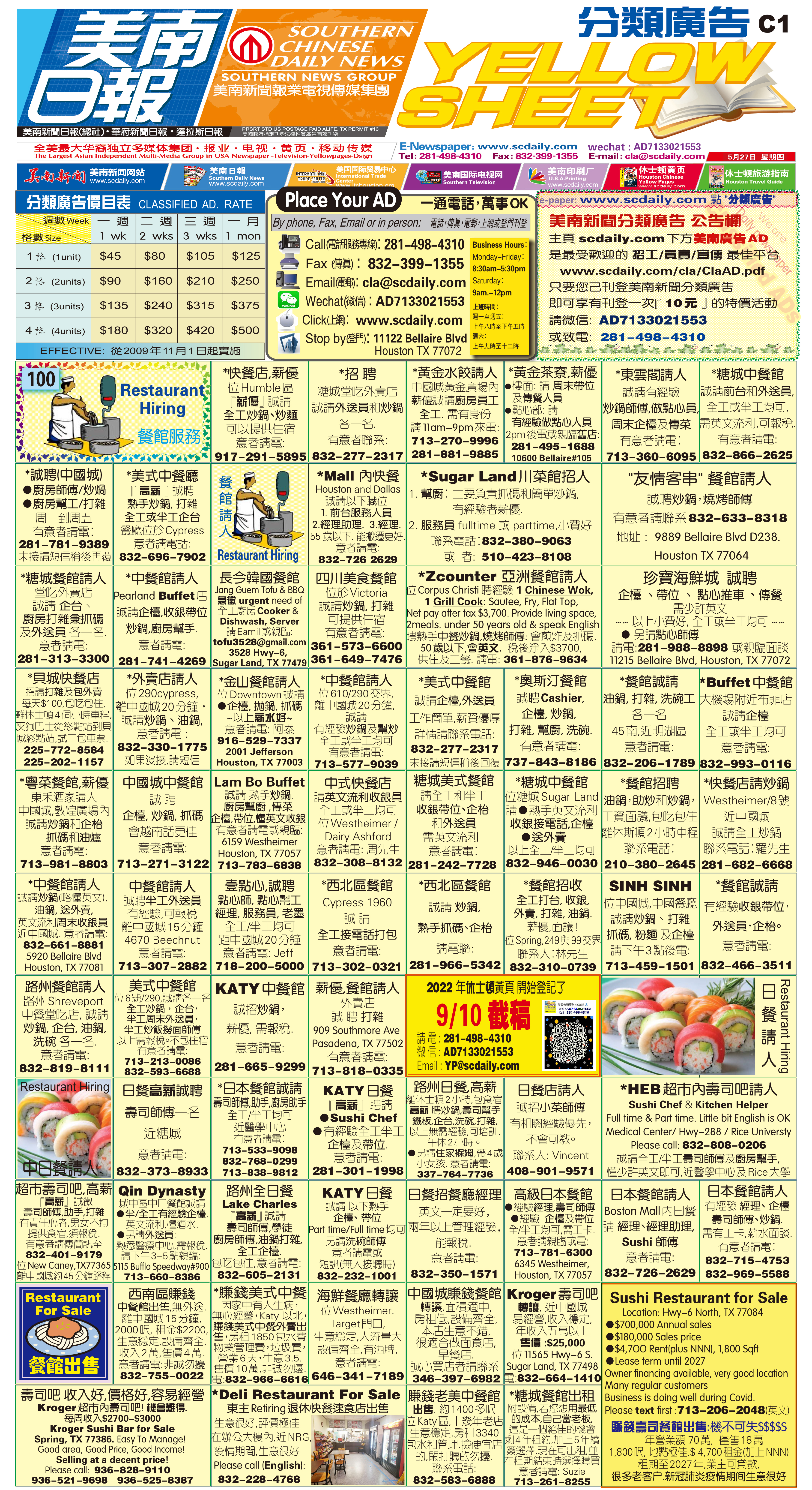210526 Epaper

 A Section B SectionC Section D Section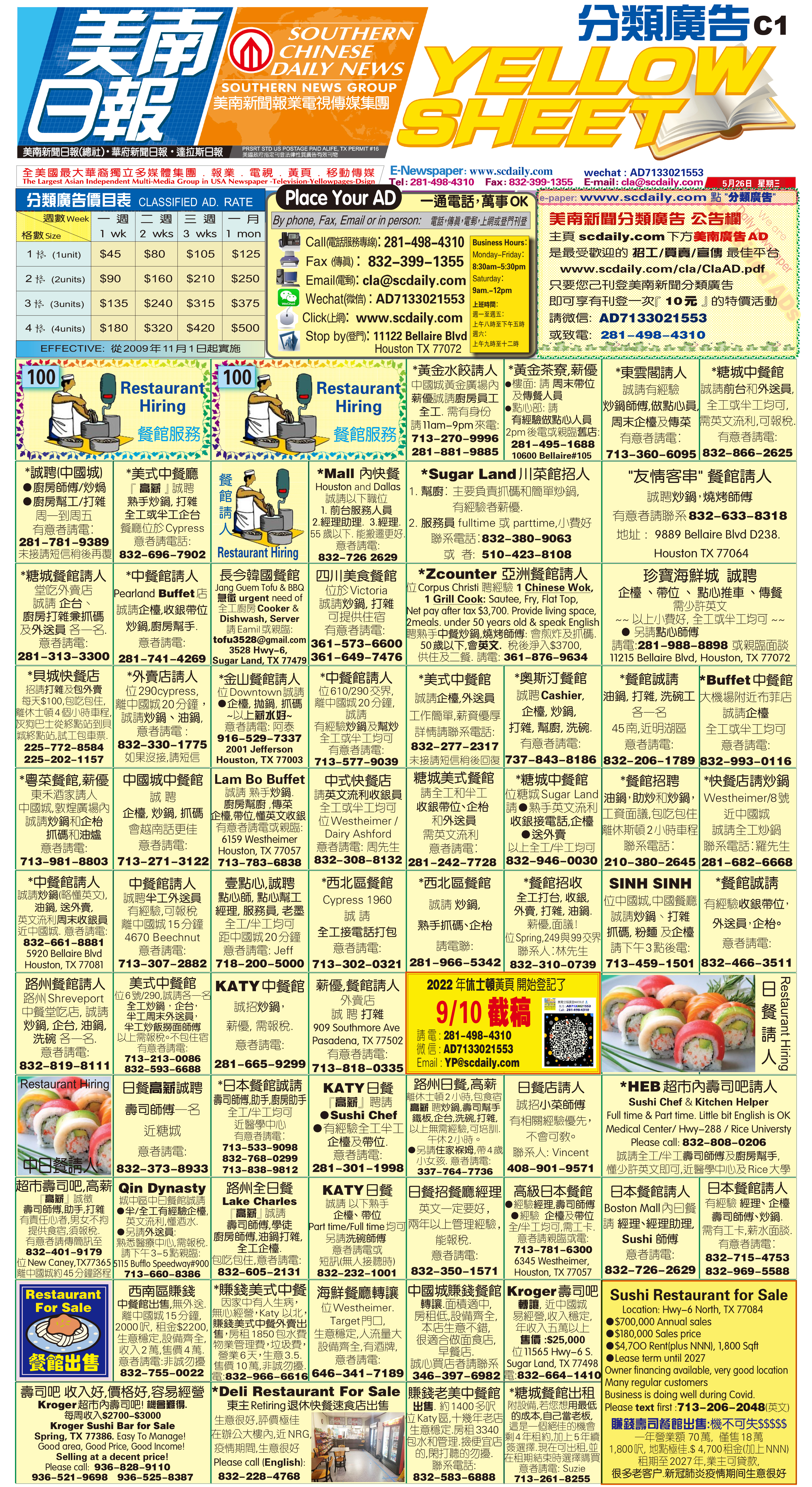210525 Epaper

 A Section B SectionC Section D Section210524 Epaper

 A Section B SectionC Section D Section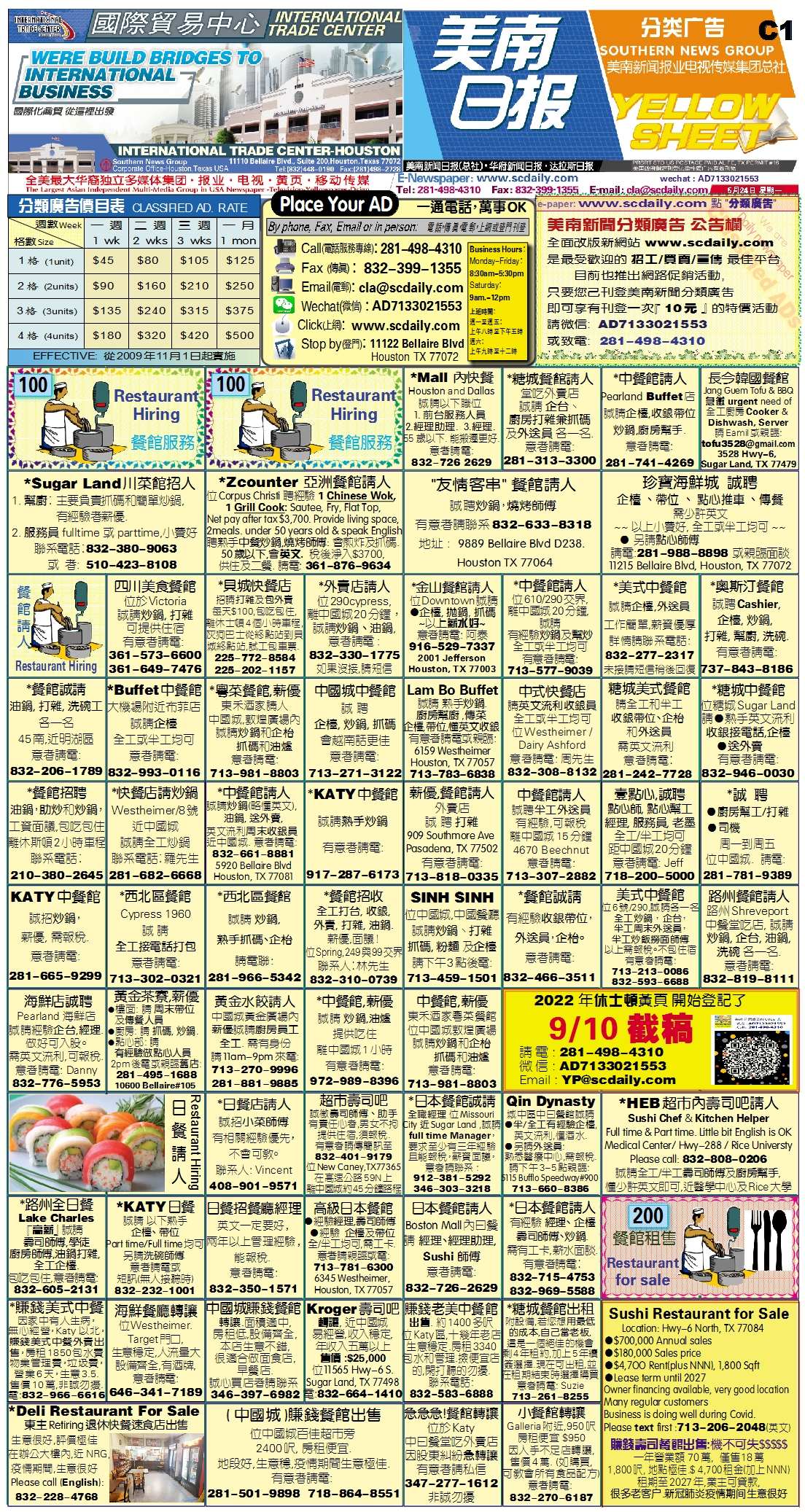210523 Epaper

 A Section B SectionC Section D Section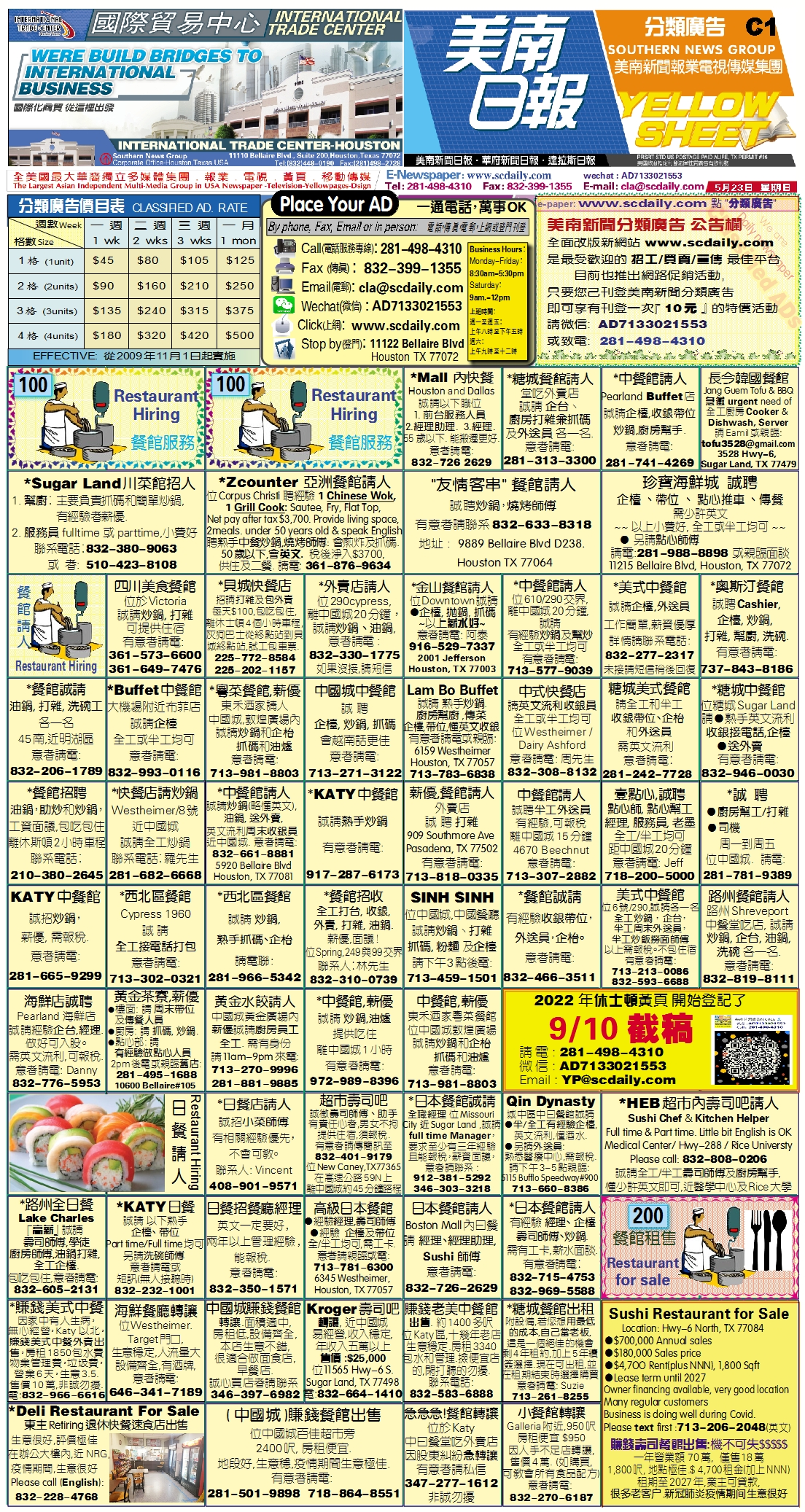210521 Epaper

 A Section B Section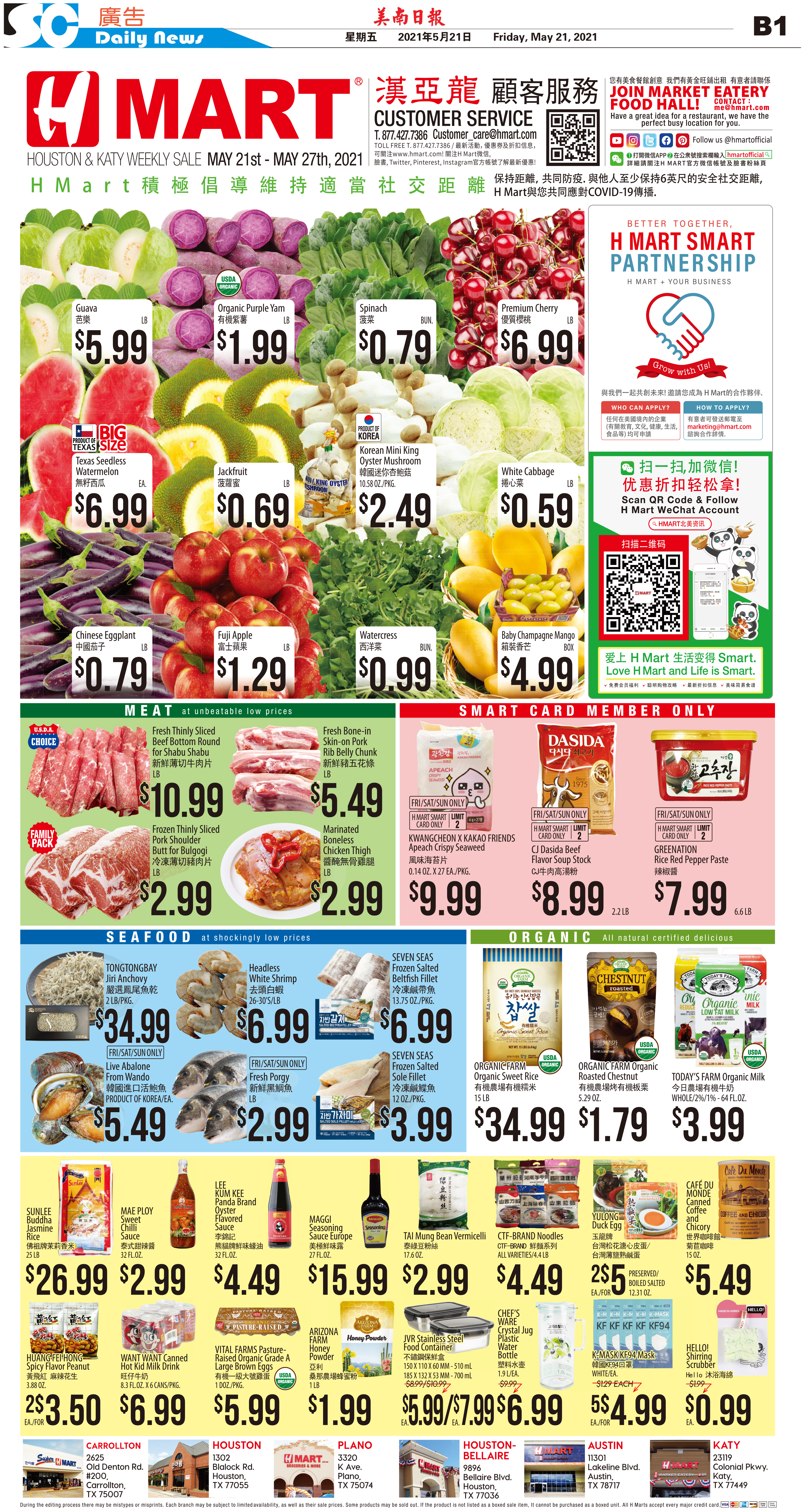C Section D Section210522 Epaper

 A Section B SectionC Section D Section210520 Epaper

 A Section B SectionC Section D Section